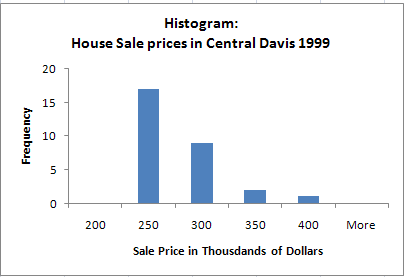## EXCEL 2007: Histogram

A. Colin Cameron, Dept. of Economics, Univ. of Calif. - Davis

This January 2009 help sheet gives information on how to

• create histograms in Excel
• variations on a histogram

DATA

We read in the following data on house sale prices in Central Davis in 1999, measured in thousands of dollars.
375, 340, 310, 279.9, 278.5, 273, 272, 270, 270, 258.5, 255, 253, 249, 245, 244, 241, 239.5, 238, 236.5, 235, 235, 233, 230, 229, 224.5, 220, 213, 212, 204.
[One way to get this data into column format is to cut the data into a text editor and manually type in enter after each observation and delete the comma].
Simplest is to open the file housepricedata.csv

HISTOGRAM

There are 29 observations. Interpretation of the data is made somewhat easier by the data already being ordered (if it is not ordered we can order it using Data Tab | Sort command). But it is useful to collapse the data further.

A histogram does this by counting the number of observations that fall within a certain range (a "bin") and then plotting this frequency against the bin value.

In Excel choose Data Tab and Data Analysis within the Analysis group.
[Note: if the Data Analysis group is not there then see Excel 2007: Access and Activating the Data Analysis Add-inThis yields the Data Analysis dialog box, which has histogram as an entry.
Highlight histogram and click okay.• For input range either type in by hand the input range (e.g. A1:A30 where A1 is a label such as Sales Price) or select the data range on the spreadsheet by clicking on the first entry (the label) and then dragging the mouse down and de-clicking at the last data entry.
• For bin range either put in nothing, in which case Excel will choose the bin range, or better still give your own bin range, explained further below.
• For labels click Labels if you have provided a label for the data (always good practice).
• In output range click and give a cell which has at least, say, 10 free cells to the right and 15 free cells below it). If the data are in column A a good choice might be, say, cell C3. Alternatively you can put the chart on a new worksheet but then it is more difficult to keep track of where the chart originated from.
• Select Chart output (but not Pareto or cumulative percentage).Hit OK. This gives the following histogram.For this data Excel has chosen six intervals with boundaries 204.0, 238.2, 272.4, 306.6, 340.8.
The general rule Excel uses is equal-width intervals with the number of intervals approximately equal to the square root of the number of data points.

For these data the number of intervals seems reasonable but the cell boundaries are not nice rounded numbers.
Rounding off to the nearest \$50,000 seems natural, so we might choose the interval boundaries as 200, 250, 300, 350 and 400.
This choice can be done by creating a bin. In, say, columns B1:B6 type, respectively House Price, 200, 250, 300, 350, 400.
(The entry in B1 will be used to automatically label the x-axis of the histogram).

In Excel choose the data Tab and Data Analysis within the Data group and then histogram and hit the histogram key.

• Provide the same outputs as earlier, which Excel should automatically display.
(change the output range to a new value if you want to keep the first histogram).
• Provide the Bin range by either type in by hand the bin range (e.g. B1:B6 where B1 is a label such as Sales Price) or select the data range on the spreadsheet by clicking on the first entry (the label) and then dragging the mouse down and de-clicking at the last bin entry.Then hit OK. We obtainNow the interval boundaries are nice rounded numbers.

Further improvements to the histogram are:
• Expand the size of the histogram by highlighting the chart area and dragging on the bottom right corner.
• Provide a clear histogram title such as House Sale Prices in Central Davis 1999 which is input by clicking on the chart title area and changing the text.
• Delete the Frequency Legend Entry on the right-hand side (here frequency) by clicking on it and hitting the delete key.
Further details on improving the presentation of graphics is given in Excel: Charts (bar, column, pie, line).

For example, consider the followingInterpretation: Excel labels the histogram by using the upper value in the interval.
Thus the frequency of 17 labeled under 250 means that there were 17 observations in the sample that took values greater than 200 and less than or equal to 250.
It would be clearer to label this interval using its midpoint of 225, and this is done by some other programs.

You can move the chart within the worksheet by clicking on it, leaving the mouse depressed, and drag the mouse.
If you need to enlarge the chart size, then resize the entire chart by putting mouse icon on bottom right hand corner of chart and dragging down to appropriate size.

VARIATIONS ON A HISTOGRAM

Variations on a histogram are

• Select Cumulative percentage to have an additional line added to the chart which shows the cumulative frequency distribution, with scale given on the right-hand side.
• Select Pareto (sorted histogram) to order the histogram categories by the frequency of occurrence. This is useful for categorical data but less useful for numerical data which are already naturally ordered.
• Select both Pareto (sorted histogram) and Cumulative percentage to get a histogram sorted by frequencuy of occurrence and the associated cumulative frequency distribution.

For further information on how to use Excel
go to http://cameron.econ.ucdavis.edu/excel/excel.html